# Introduction to Geospatial Data in Python

In this tutorial, you will use geospatial data to plot the path of Hurricane Florence from August 30th to September 18th.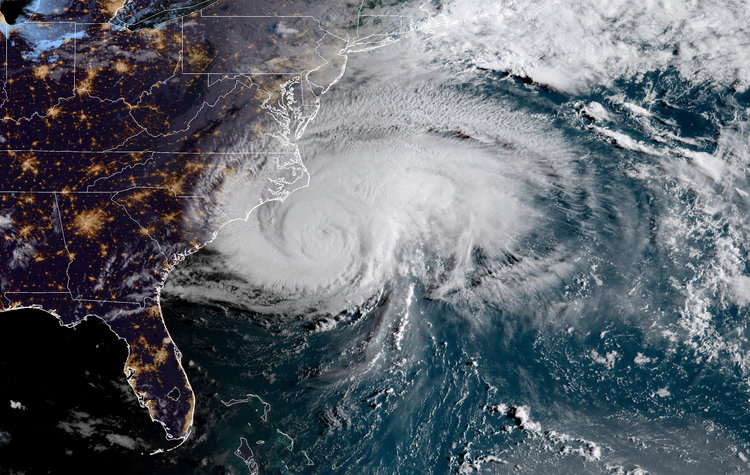In this tutorial, you will get to know the two packages that are popular to work with geospatial data: geopandas and Shapely. Then you will apply these two packages to read in the geospatial data using Python and plotting the trace of Hurricane Florence from August 30th to September 18th.

## What is Geospatial Data?

Spatial data, Geospatial data, GIS data or geodata, are names for numeric data that identifies the geographical location of a physical object such as a building, a street, a town, a city, a country, etc. according to a geographic coordinate system. From the spatial data, you can find out not only the location but also the length, size, area or shape of any object. An example of a kind of spatial data that you can get are: coordinates of an object such as latitude, longitude, and elevation. Geographic Information Systems (GIS) or other specialized software applications can be used to access, visualize, manipulate and analyze geospatial data.

## Why Geospatial Data?

Geospatial data has a vast number of applications in our daily life. One example is the Maps application that you use to navigate from one location to the others. Another one that you may see every day is on the weather channel.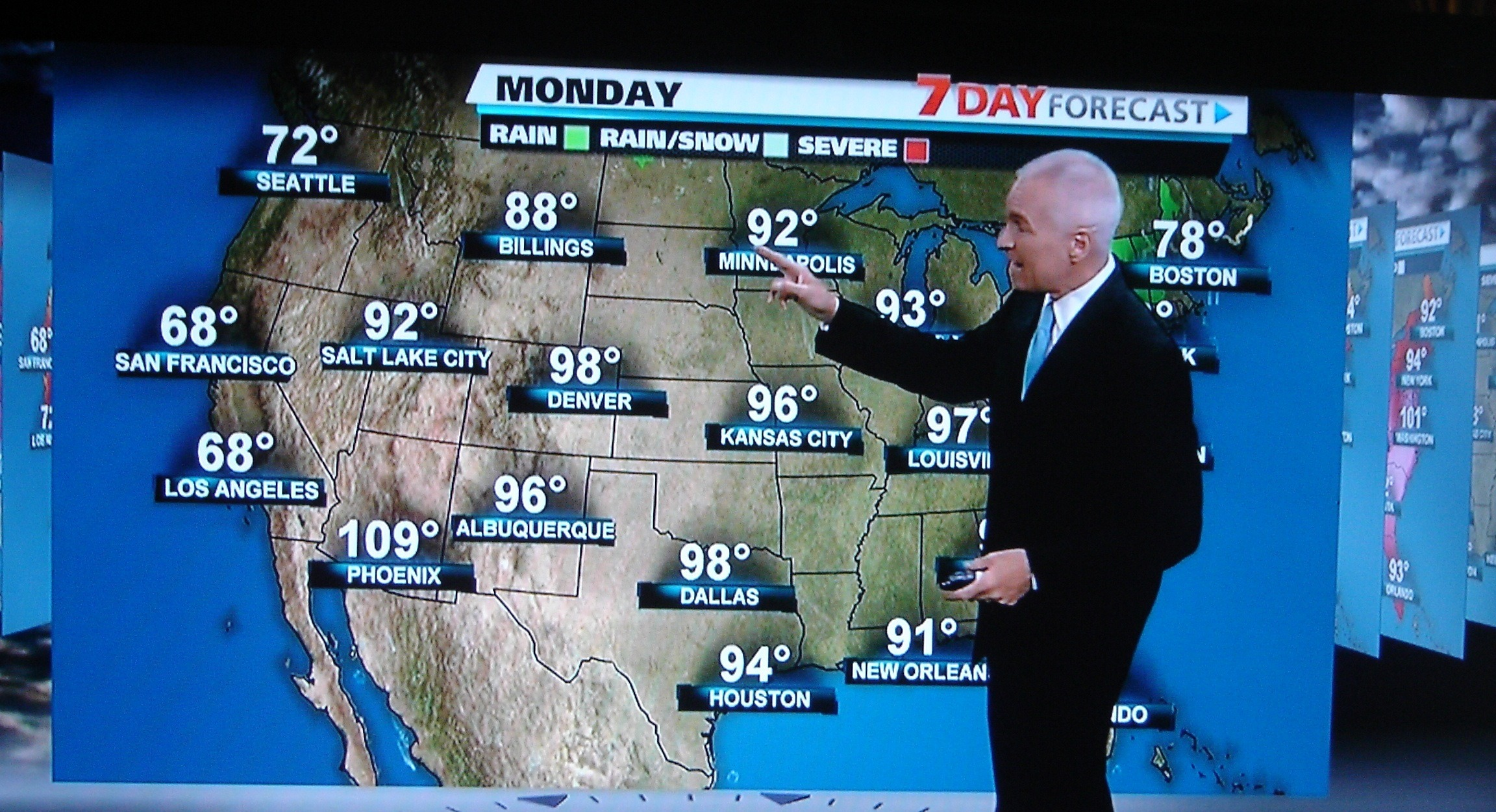Yes, geospatial data is used to represent position information of something with respect to other things around it: your house on the city map, the hurricane on the world map and so on. So in this tutorial, you will take a look at the destructive hurricane Florence and track its location.

### Packages requirements

Don't skip ahead. This is an important part. If you are not sure you've satisfied the requirements, just check again. First and foremost, you will need to have all the packages below installed.

• Pandas: provide data structures and data analysis tools
• Numpy: a fundamental package for scientific computing with Python
• SciPy:(pronounced “Sigh Pie”) is a Python-based ecosystem of open-source software for mathematics, science, and engineering
• RTree: a ctypes Python wrapper of libspatialindex that provides a number of advanced spatial indexing features
• GDAL: translator library for raster and vector geospatial data formats
• Fiona: Fiona reads and writes spatial data files
• Shapely: Geometric objects, predicates, and operations
• GeoPandas: extends the datatypes used by pandas to allow spatial operations on geometric types.
• PySAL: a library of spatial analysis functions written in Python intended to support the development of high-level applications.
• Matplotlib: Python 2D plotting library
• Missingno: Missing data visualization module for Python

Install all the packages following the order above to ensure everything worked. Some packages are pre-requisites for the others, for example: to install `GeoPandas`, it requires `Shapely`, and to install `Shapely`; `RTree`, `GDAL`, and `Fiona` should be installed. The easiest way to install a package is: `pip install PACKAGE_NAME`

If you are using Windows and can't install the package that way, check this website to download the related packages and do `pip install PATH_TO_PACKAGE`.

As you may know, the terrifying hurricane Florence just passed through part of the East Coast of the United States, leaving an estimated damage of 17 billion USD. This tutorial will help you to find out where it came from, when and where it got stronger and understand more about this natural disaster and analyze it in Python. There are many websites providing information about this hurricane. For example, this website provided data for several storms and hurricanes in the States from 1902 to 2018, so there is a ton of available data for you to work later on. For this tutorial, you will only use the Hurricane Florence data

You will also use the US map geospatial data from the internet. This blog post by Eric Celeste has all kind of boundary data files for US counties and states. The data used in this tutorial is the US States, 5m, GeoJSON file.

`````` # Load all importance packages
import geopandas
import numpy as np
import pandas as pd
from shapely.geometry import Point

import missingno as msn

import seaborn as sns
import matplotlib.pyplot as plt

% matplotlib inline``````

First, let's look at the first geospatial dataframe: US States Geodata

``````# Getting to know GEOJSON file:
``````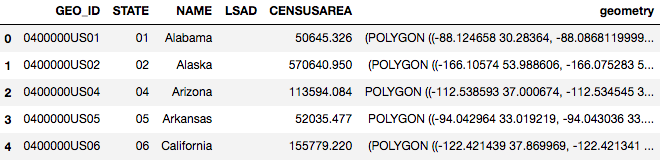Checking the type of the dataframe that you just load in, you can see that it's Geo Data Frame, which has all the regular characteristics of a Pandas DataFrame.

``````type(country)
``````
``````geopandas.geodataframe.GeoDataFrame
``````

Checking the data type of the column containing coordinates: it's GeoSeries.

``````type(country.geometry)
``````
``````geopandas.geoseries.GeoSeries
``````

Each value in the GeoSeries is a Shapely Object. It can be:

• Point
• Line
• Polygon
• MultiPolygon

Each object can be used for a different type of physical object such as: Point for building, Line for Street, Polygon for city, and MultiPolygon for country with multiple cities inside. For more information about each Geometric object, read this article: https://shapely.readthedocs.io/en/stable/manual.html#geometric-objects

``````type(country.geometry)
``````
``````shapely.geometry.multipolygon.MultiPolygon
``````

Similar to a Pandas DataFrame, a GeoDataFrame also has attribute plot, which makes use of the geometry character within the dataframe to plot a map:

``````country.plot()
``````
``````<matplotlib.axes._subplots.AxesSubplot at 0x1cfe68c1358>
``````As you may see, the US map is relatively small compared to the frame. It's because the information includes Alaska, Hawaii and Puerto Rico, which spread out around. For this tutorial purpose, you can exclude Alaska and Hawaii as the hurricane did not go anywhere near those two states. You can also add the figure size and color to customize your own plot:

``````# Exclude Alaska and Hawaii for now
``````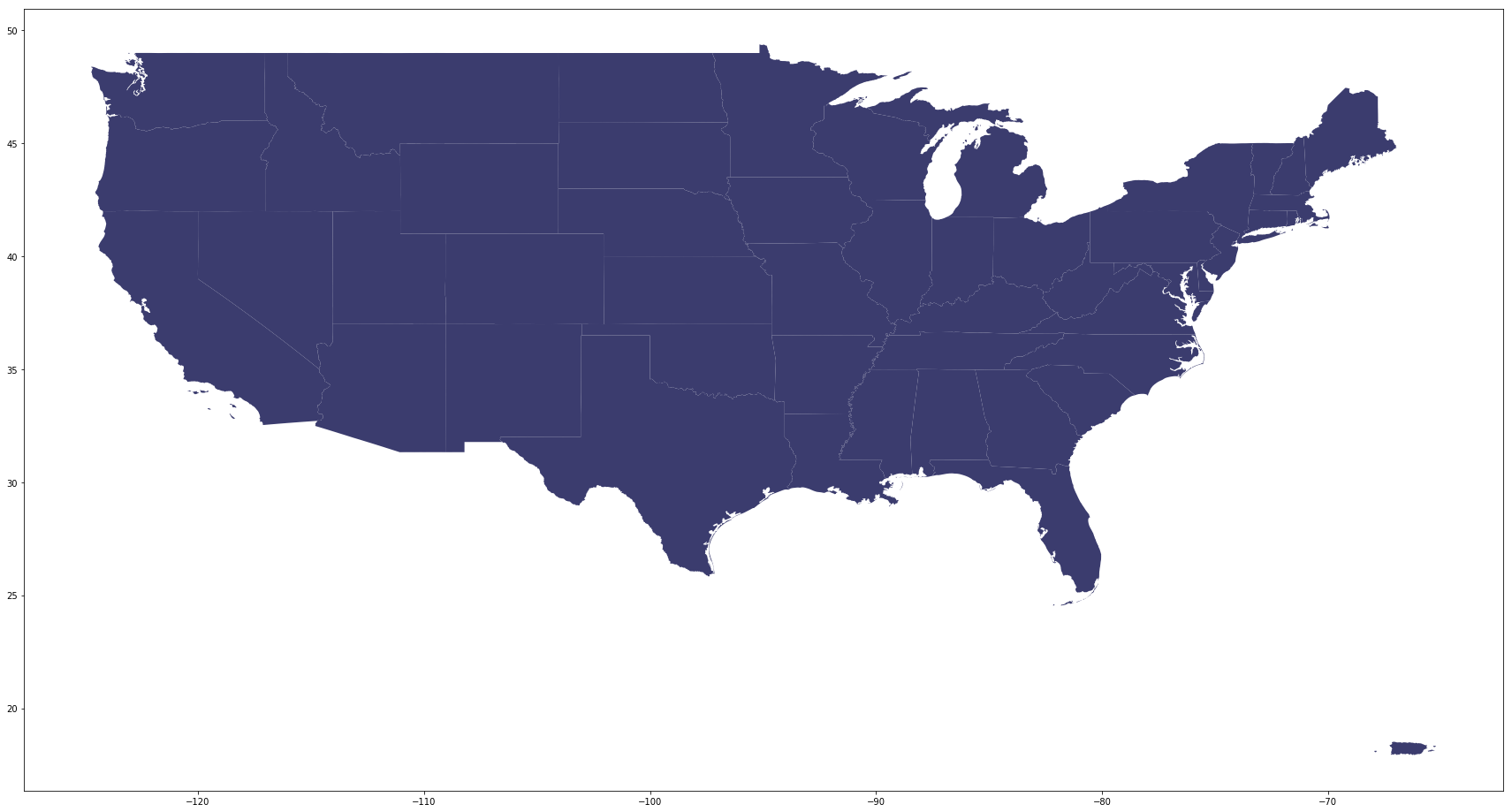Not so hard, right! Now you have the US map, let's load in hurricane data:

``````florence = pd.read_csv('data/florence.csv')
``````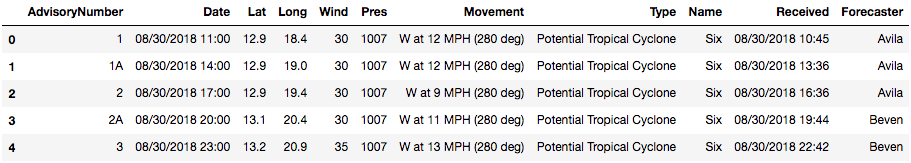## Exploratory Data Analysis

This is always the first thing you do when loading any dataset:

• Checking the information, data type
• Any missing value
• Statistical data
``````florence.info()
``````
``````<class 'pandas.core.frame.DataFrame'>
RangeIndex: 105 entries, 0 to 104
Data columns (total 11 columns):
Date              105 non-null object
Lat               105 non-null float64
Long              105 non-null float64
Wind              105 non-null int64
Pres              105 non-null int64
Movement          105 non-null object
Type              105 non-null object
Name              105 non-null object
Forecaster        104 non-null object
dtypes: float64(2), int64(2), object(7)
memory usage: 9.1+ KB
``````

Checking missing values using the missingno package. This is a useful package using visualization to show missing data. As you can see below, there's only one missing value in the column "Forecaster" which you don't need for this tutorial. So you can ignore it for now.

``````# Notice you can always adjust the color of the visualization
msn.bar(florence, color='darkolivegreen');
``````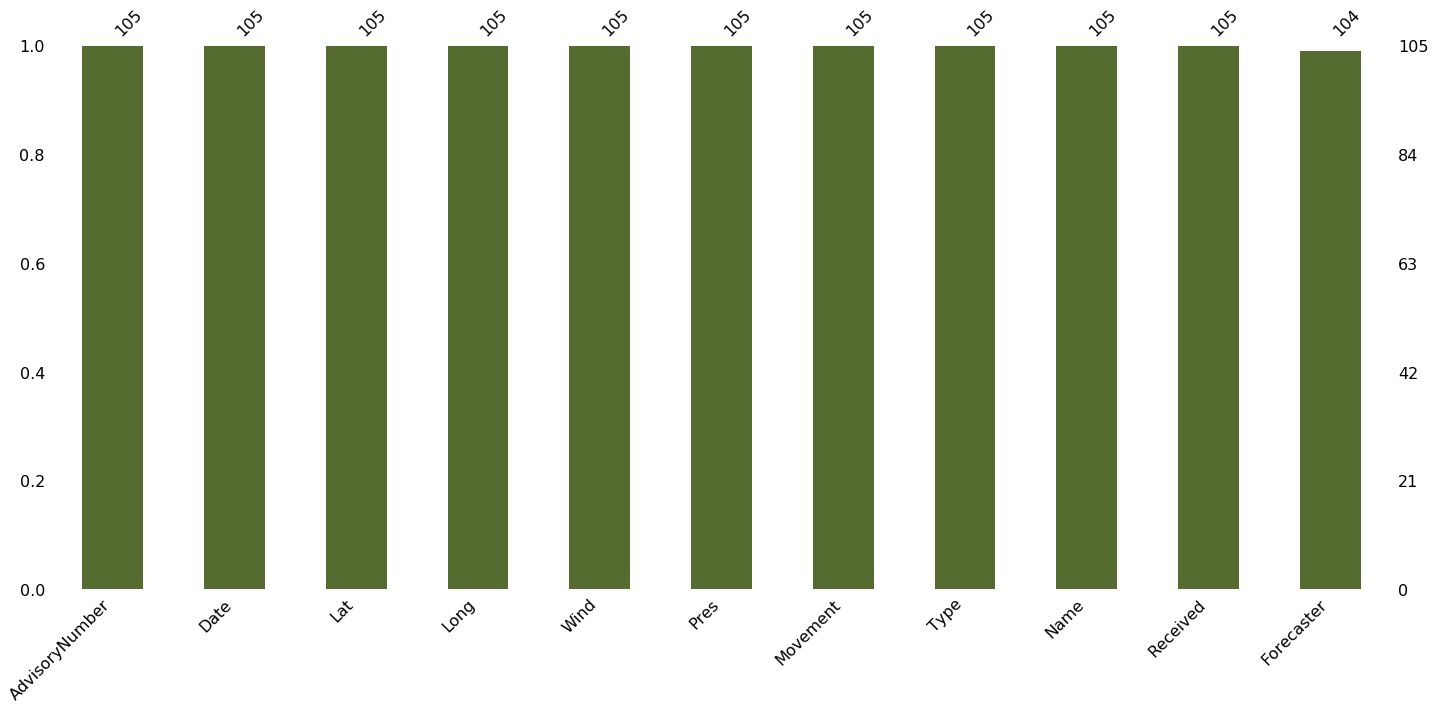Take a look at some statistical information, some could be very useful such as mean wind speed, maximum and minimum wind speed of this hurricane, etc.

``````# Statistical information
florence.describe()
``````
Lat Long Wind Pres
count 105.000000 105.000000 105.000000 105.000000
mean 25.931429 56.938095 74.428571 981.571429
std 7.975917 20.878865 36.560765 22.780667
min 12.900000 18.400000 25.000000 939.000000
25% 18.900000 41.000000 40.000000 956.000000
50% 25.100000 60.000000 70.000000 989.000000
75% 33.600000 76.400000 105.000000 1002.000000
max 42.600000 82.900000 140.000000 1008.000000

For most data, you will need to clean up and take only what you need to work on. Here, you only need the time, the coordinates: latitude and longitude, Wind speed, Pressure, and Name. Movement and Type are optional, but the rest could be dropped.

``````# dropping all unused features:
``````Normally, if you plot the data by itself, there is no need to take extra care for the coordinate. However, if you want it to look similar to how you look on the map, it's important to check on the longitude and latitude. Here the longitude is west, you will need to add "-" in front of the number to correctly plot the data:

``````# Add "-" in front of the number to correctly plot the data:
florence['Long'] = 0 - florence['Long']
``````Then you can combine Lattitude and Longitude to create hurricane coordinates, which will subsequently be turned into GeoPoint for visualization purpose.

``````# Combining Lattitude and Longitude to create hurricane coordinates:
florence['coordinates'] = florence[['Long', 'Lat']].values.tolist()
``````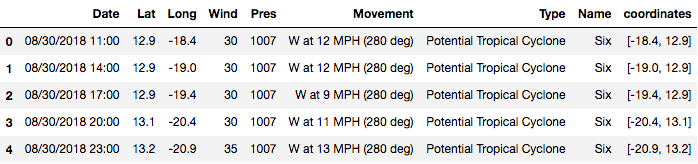``````# Change the coordinates to a geoPoint
florence['coordinates'] = florence['coordinates'].apply(Point)
``````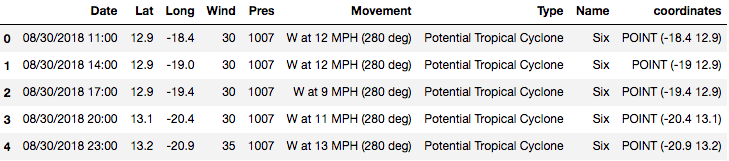Checking the type of the `florence` dataframe and column `coordinates` of `florence` data. It's pandas DataFrame and pandas Series.

``````type(florence)
``````
``````pandas.core.frame.DataFrame
``````
``````type(florence['coordinates'])
``````
``````pandas.core.series.Series
``````

After converting the data into geospatial data, we will check the type of `florence` dataframe and column `coordinates` of Florence data again. Now it's Geo DataFrame and GeoSeries.

``````# Convert the count df to geodf
florence = geopandas.GeoDataFrame(florence, geometry='coordinates')
````````````type(florence)
``````
``````geopandas.geodataframe.GeoDataFrame
``````
``````type(florence['coordinates'])
``````
``````geopandas.geoseries.GeoSeries
``````

Notice that even though it's now a Geo DataFrame and Geo Series, it still behaves like a normal DataFrame and a Series. This means you can still perform filtering, groupby for the dataframe or extract the min, max, or mean values of the column.

``````# Filtering from before the hurricane was named.
florence[florence['Name']=='Six']
````````````# Groupping by name to see how many names it has in the data set:
florence.groupby('Name').Type.count()
``````
``````Name
FLORENCE     6
Florence    85
SIX          4
Six         10
Name: Type, dtype: int64
``````

Finding the mean wind speed of hurrican Florence:

``````print("Mean wind speed of Hurricane Florence is {} mph and it can go up to {} mph maximum".format(round(florence.Wind.mean(),4),
florence.Wind.max()))
``````
``````Mean wind speed of Hurricane Florence is 74.4286 mph and it can go up to 140 mph maximum
``````

So the average wind speed of hurricane Florence is 74.43 miles per hour (119.78 km per hour) and the maximum is 140 miles per hour (225.308 km per hour). To imagine how scary this wind speed is, the website Beaufort Wind Scale, developed by U.K Royal Navy, shows the appearance of wind effects on the water and on land. With the speed of 48 to 55 miles per hours, it can already break and uproot trees, and cause "considerable structural damage".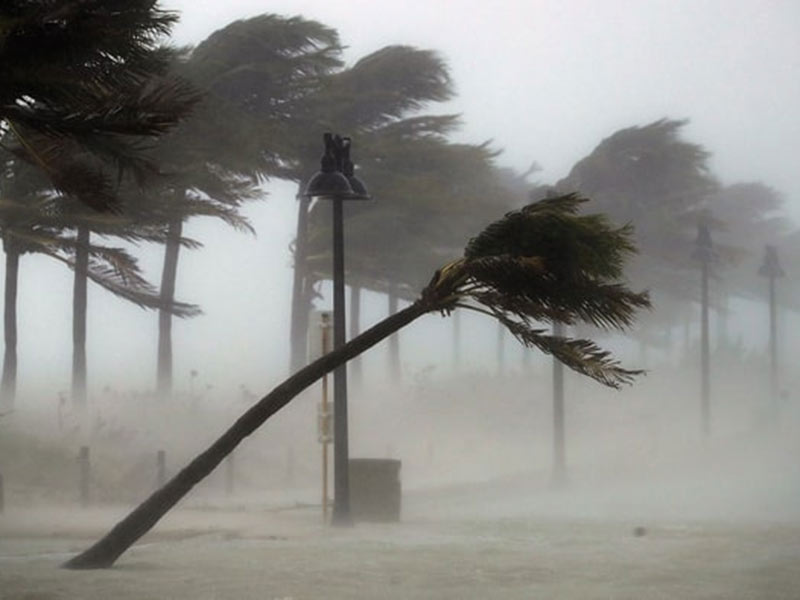You don't want to be there at this moment.

## Visualization

Similar to pandas Dataframe, a GeoDataFrame also has `.plot` attribute. However, this attribute makes use of the coordinate within the GeoDataFrame to map it out. Let's take a look:

``````florence.plot(figsize=(20,10));
``````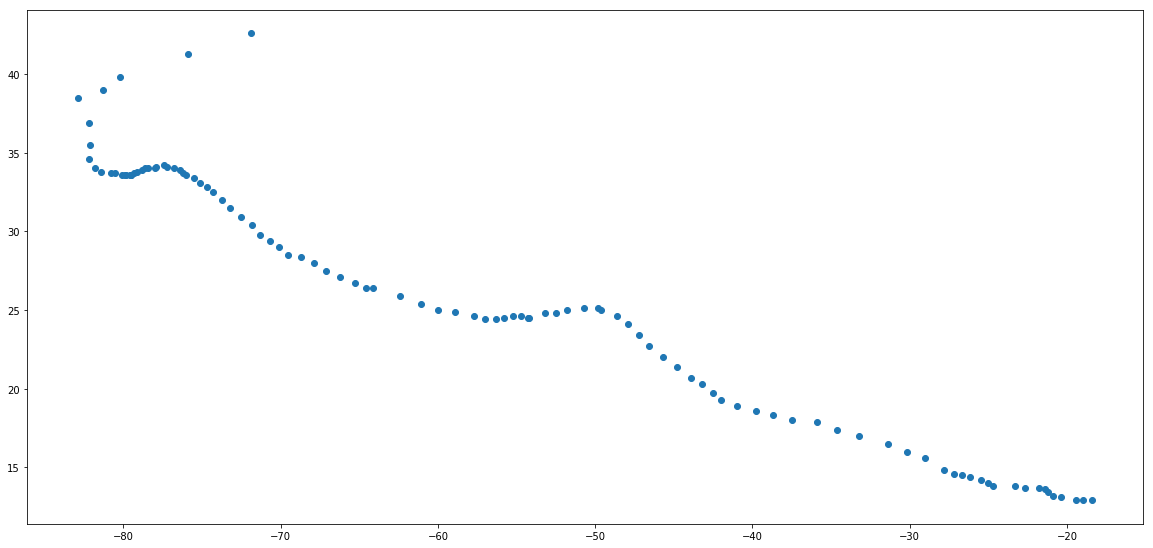What happened? All you can see is a bunch of points with no navigation. Is there anything wrong?
No, it's all fine. Because this dataframe only have coordinates information (location) of hurricane Florence at each time point, we can only plot the position on a blank map.

So, the next step is plotting the hurricane position on the US map to see where it hit and how strong it was at that time. To do so, you will use the US map coordinates (data we loaded in the beginning) as the base and plotting hurricane Florence position on top of it.

`````` # Plotting to see the hurricane overlay the US map:
fig, ax = plt.subplots(1, figsize=(30,20))
base = country[country['NAME'].isin(['Alaska','Hawaii']) == False].plot(ax=ax, color='#3B3C6E')

# plotting the hurricane position on top with red color to stand out:
florence.plot(ax=base, color='darkred', marker="*", markersize=10);``````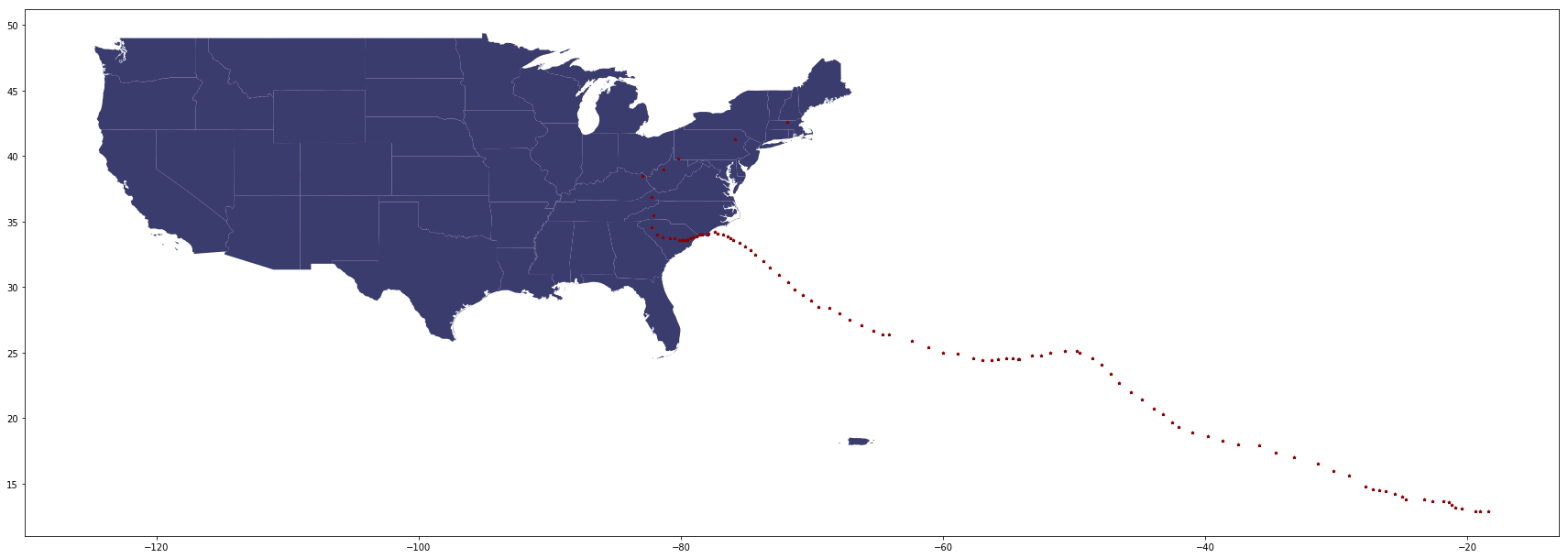Looks great! Now, we will finish it with more details such as:

• Color the hurricane position based on the wind speed to see how strong the hurricane was when it hit each city.
• Remove axis
• Saving the result to an image file to use later
`````` fig, ax = plt.subplots(1, figsize=(20,20))
base = country[country['NAME'].isin(['Alaska','Hawaii']) == False].plot(ax=ax, color='#3B3C6E')
florence.plot(ax=base, column='Wind', marker="<", markersize=10, cmap='cool', label="Wind speed(mph)")
_ = ax.axis('off')
plt.legend()
ax.set_title("Hurricane Florence in US Map", fontsize=25)
plt.savefig('Hurricane_footage.png',bbox_inches='tight');``````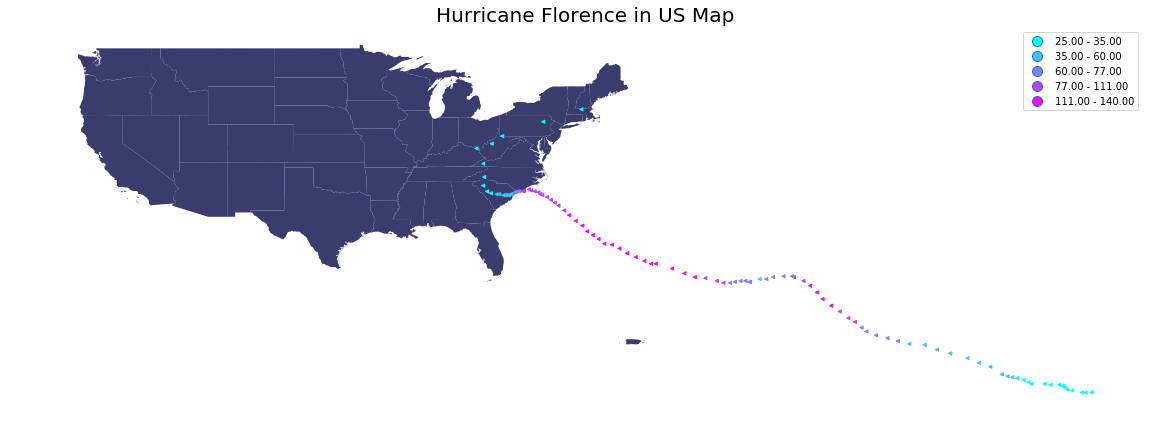So the hurricane was strongest when it's offshore near the east coast. As it approached the land, the hurricane started losing its strength, but at the wind speed in range 60 to 77 miles per hour, it can still make horrible damages.

## Conclusion

Great work! You have learned some necessary steps to work with geospatial data in Python. You also learn how to plot the geospatial data and customize the shape, color, and overlay of plots to show a story. If you want to practice your skills, there is a ton of geospatial data available online for you to try your hand on. One example is the weather website that I provided above.

For sources, please take a look at this book: “Python Geospatial Development Essentials” by Karim Bahgat (2015) for deeper guidance.

If you'd like to get in touch with me, you can drop me an e-mail at [email protected] or connect with me via LinkedIn. Have fun learning and stay safe!

If you would like to learn more about geospatial data in Python, take DataCamp's Visualizing Geospatial Data in Python course.

Below is the data used in this tutorial: